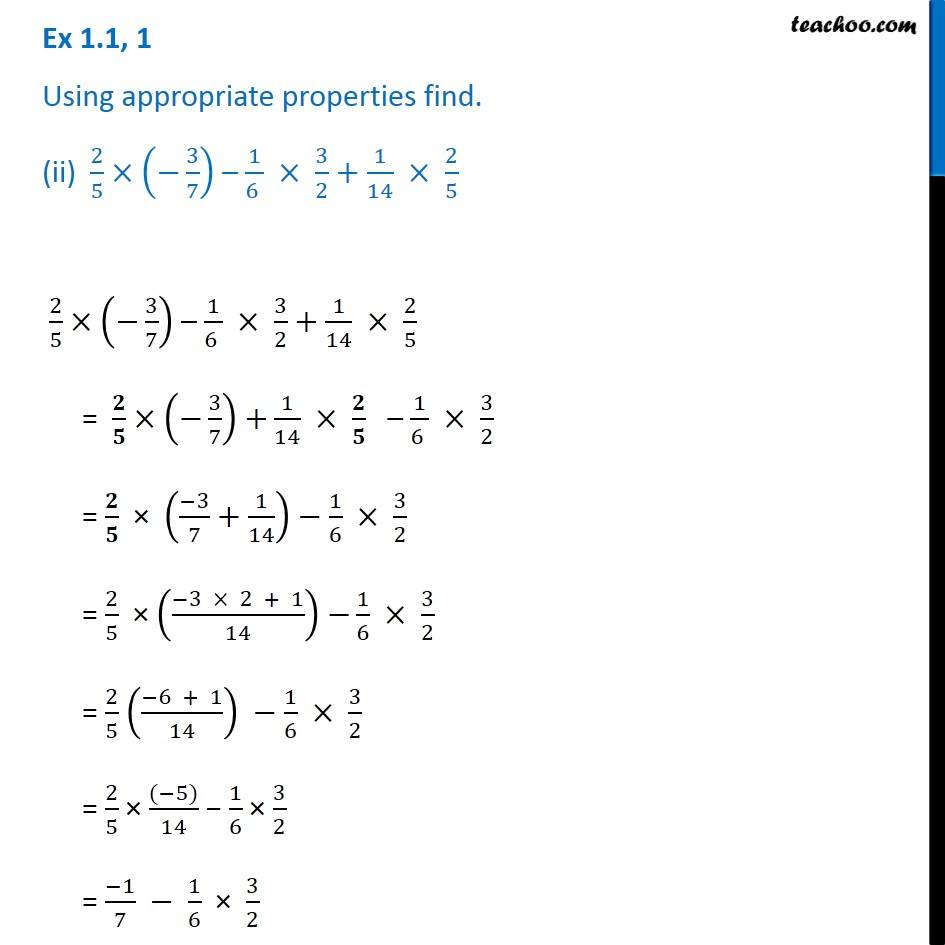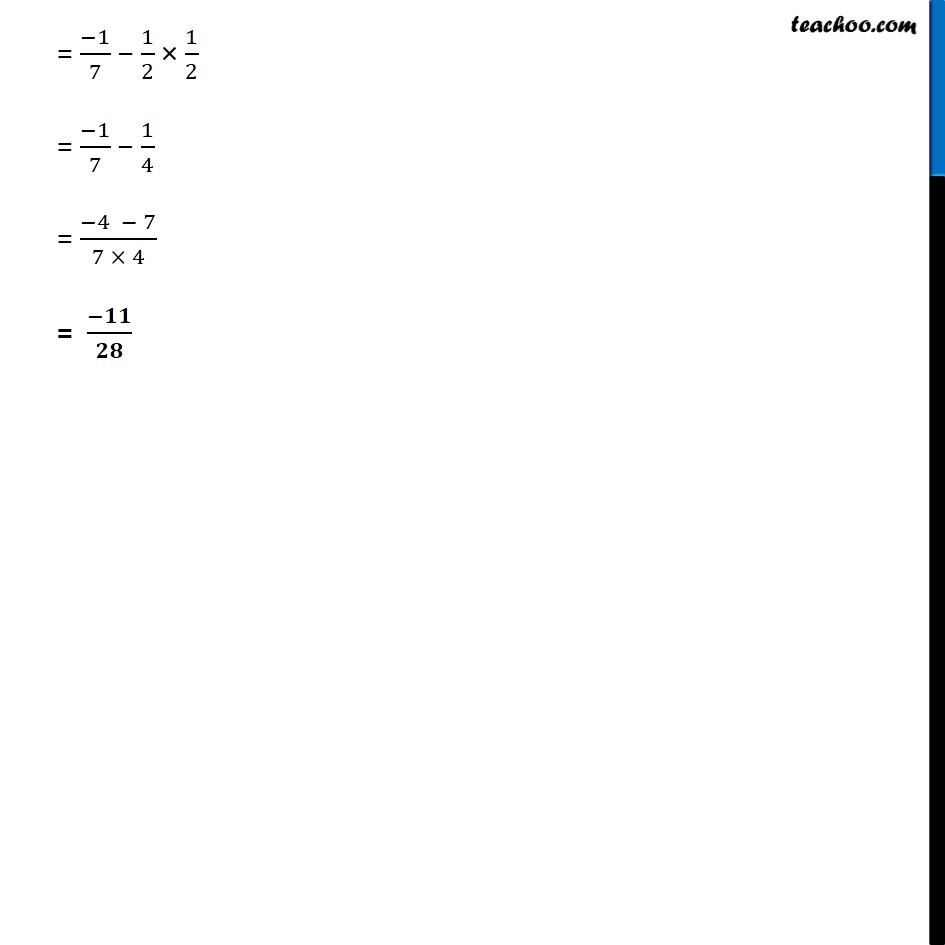Ex 1.1

Chapter 1 Class 8 Rational Numbers
Serial order wiseLearn in your speed, with individual attention - Teachoo Maths 1-on-1 Class

### Transcript

Ex 1.1, 1 Using appropriate properties find. (ii) 2/5×(−3/7) – 1/(6 ) × 3/2+1/14 × 2/5 2/5×(−3/7) – 1/(6 ) × 3/2+1/14 × 2/5 = 𝟐/𝟓× (−3/7) +1/14 × 𝟐/𝟓 – 1/(6 ) × 3/2 = 𝟐/𝟓 × ((−3)/7+1/14)−1/6 × 3/2 = 2/5 × ((−3 × 2 + 1)/14) −1/6 × 3/2 = 2/5 ((−6 + 1)/14) −1/6 × 3/2 = 2/5 × ((−5))/14 − 1/6 × 3/2 = (−1)/7 − 1/6 × 3/2 = (−1)/7 − 1/2 × 1/2 = (−1)/7 − 1/4 = (−4 − 7)/(7 × 4) = (−𝟏𝟏)/𝟐𝟖